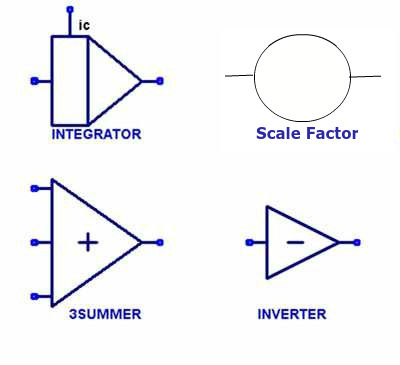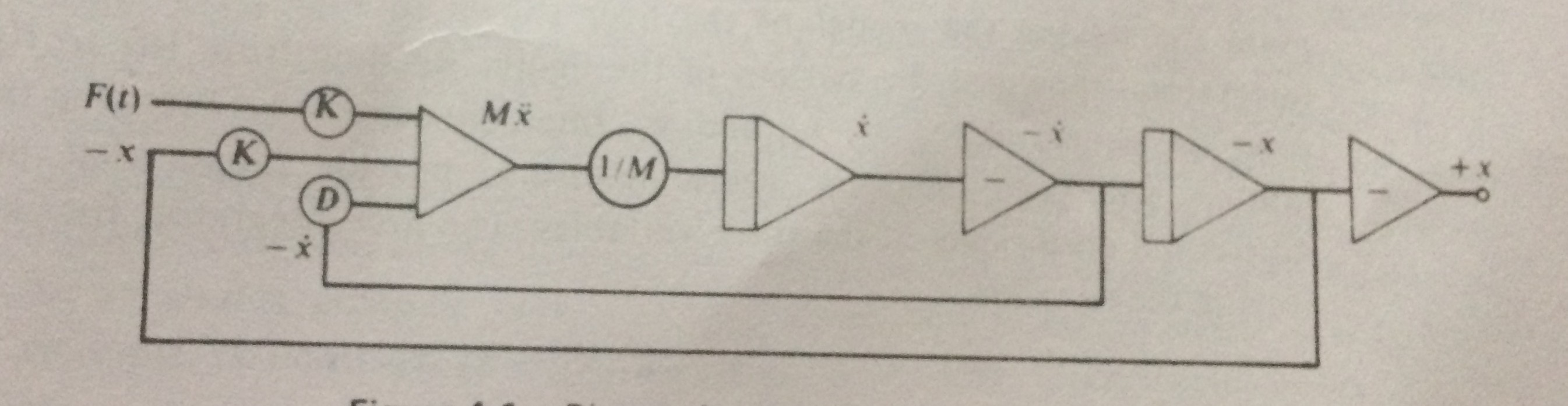### Notes of Simulation and Modelling [CT 753]

#### Continuous system simulation

Differential and Partial Differential Equations

- The equation that consists of the higher order derivatives of the dependent variable is known as differential equations.
- The differential equation is said to be linear if any of the dependent variables and its derivatives have power of one and are multiplied by the constant.
Eg: M x’’ + D x’ + K x = K F(t)
where, M, D and K are constants; F(t) is the input to the system depending upon the independent variable t; x’’ and x’ are second and first order derivatives of dependent variable x.
- The differential equation is said to be non-linear if the dependent variable or any of its derivatives are raised to a power or are combined in other way like multiplication.

- The differential equation is said to be partial if more than one independent variables occur in a differential equation.
- Eg: Equation of flow of heat in three dimensional body. It consists of four independent variables ( three dimensions and time ) and one dependent variable ( temperature ).

Necessity of differential equations:

1. Most physical and chemical process occurring in the nature involves rate of change, which requires differential equations to provide mathematical model.
2. It can be used to understand general effects of growth trends as differential equations can represent a growth rate.

Continuous System Models

- A continuous system is the system in which the activities of the main elements of the system cause smooth changes in the attributes of the entities of the system.
- On mathematical modeling, the attributes of the system are controlled by a continuous functions.
- In such system, the relationships depicts the rates at which the attributes changes.
- The continuous system is modeled using the differential equations.
- The complex continuous system with non-linearity can be simulated by showing the application to models for linear differential equations to obtain constant coefficients and then generalize to more complex equations.

Analog Computers

- Analog computers are those computers that are unified with devices like adder and integral so as to simulate the continuous mathematical model of the system, which generates continuous outputs.
- The electronic analog computer based on the use of high gain dc amplifiers are widely used analog computer.
- In such analog computer, voltages are equated to mathematical variables and the op amps can add and integrate the voltages.
- The proper configurations can handle addition of several input voltages each representing the input variables.
- The coefficient of the model equations are obtained by using the scale factors.
- The circuit can be arranged to produce integrator that provides integral w.r.t time of a single input voltage or sum of input voltages.
- Sign inverter is used to reverse the sign of the input as per the requirement of the model equation.
- The analog computer provides limited accuracy because op amps have many assumptions which can never be true in reality.

Analog Methods

- The general method to apply analog computers for the simulation of continuous system models involves following components:Automobile Suspension Problem : Example

The model is defined by the second order differential equation as:
M x’’ + D x’ + K x = K F(t)
=>M x’’ = K F(t) – D x’ - K x

The diagram analog method modeling is shown below:Hybrid Simulation

- In reality, the system is of neither a pure continuous nor a pure discrete nature.
- For simulating such system, the combination of analog and digital computers are used. Such setup is known as hybrid computers.
- The simulation provided by the hybrid computers is known as hybrid simulation.
- The major difficulty in use of hybrid simulation is that it requires high speed converters to transform signals from analog to digital form and vice versa.

Digital Analog Simulator

- Digital Analog simulators indicates the use of programming languages in digital computer to simulate the continuous system.
- The language is composed of macro-instructions which are able to act as adder, integrator and sign-changer.
- A program is written to link these macro-instructions as per the necessity which are provide virtual connections of op amps in analog computers.